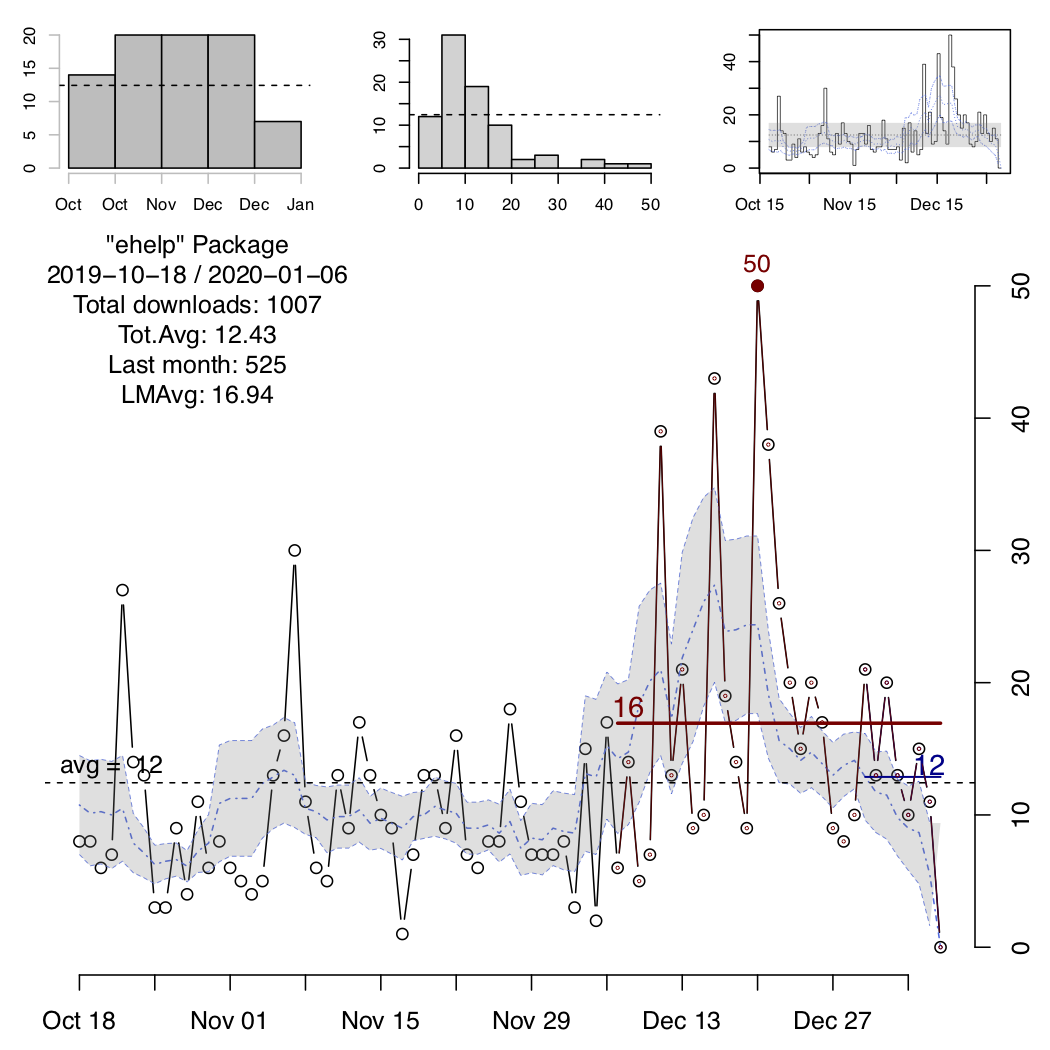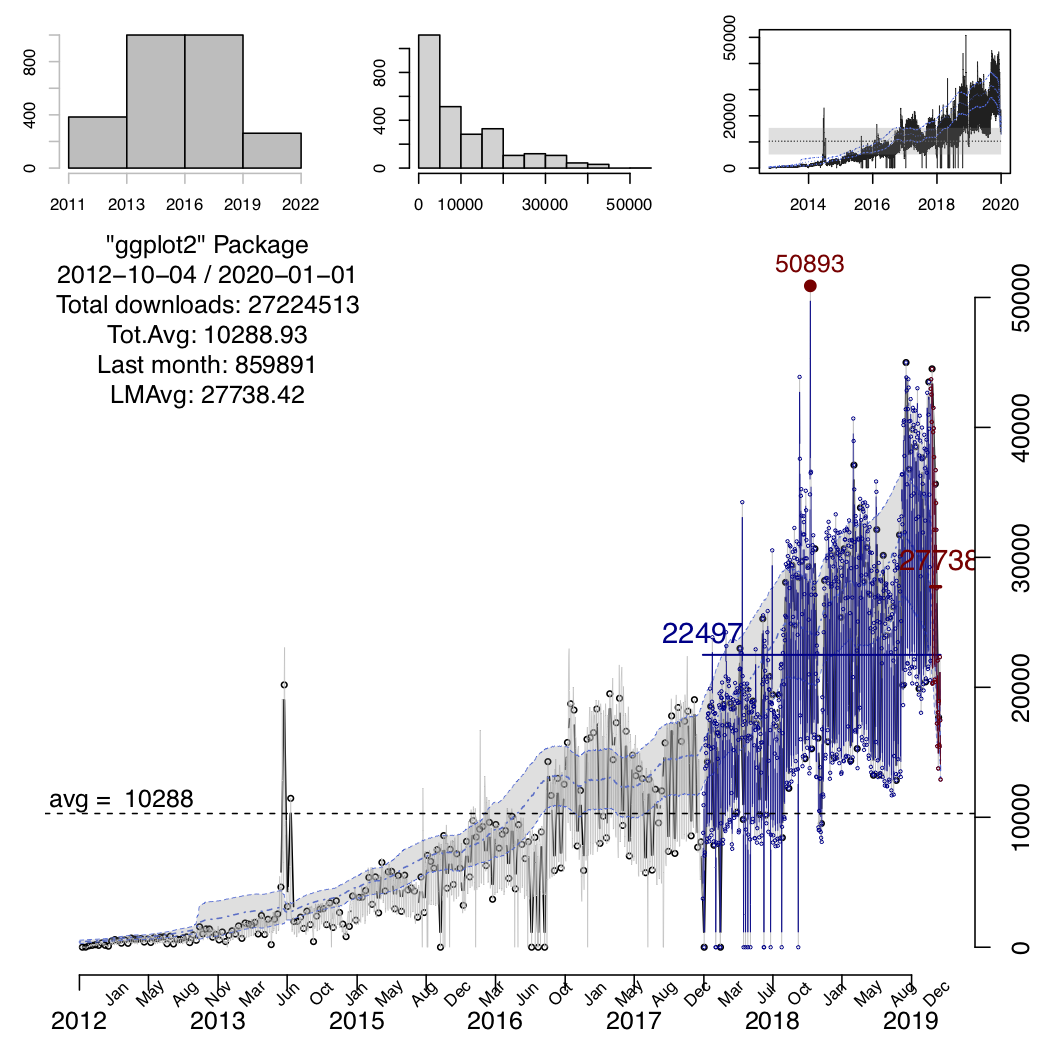## Introduction

This package allows you to visualize the number of downloads for an specific package in the CRAN repository.

The user can specify different ways to display the information: in a classic (static) plot, an interactive representation, and/or a combined figure comparing multiple packages.

## Features

### Graphical and Statistical Outcomes

• Static and interactive representations
• Comparison plots for multiple packages
• In screen output of statistics for different ranges of time

### Automatic date specification and selection

The user can specify the range of dates to be processed, however the main function of the package will run a couple of checks and adjustments on these:

1. if no dates are specified it will assume the current date as the end of the period and a year before as the starting date, ie. a period of a year since today;

2. given a range of dates, it will reset the range to the first reported download within the specified dates, so that dates previous to any reported download from the CRAN logs are not shown, in this way the package can generate a cleaner and more meaningful visualization.

### Displaying “moving” statistical estimators

In order to show a closer trend to the time series data of downloads, the package will also display moving avera ges and moving intervals of confidence. The confidence interval will be shaded in the main plot.

Both features can be turned off, using the corresponding flags in the options: `"noMovAvg"` and `"noConfBands"`.

The moving estimators (average and confidence intervarls) are comoputed using a default of 10 windows to be considered over the indicated period of time, i.e. in a given period of time the algorithm will select 10 windows resulting in an effective size for the moving window of the time range divived by 10. The confidence interval is determined using the “moving” standard deviation, ie. the standard deviation computed on the moving window. The upper limit of the confidence band is determined by +half standard deviation and the lower band by -half standard deviation in the corresponding window.

## Implementation

Visualize.CRAN.Downloads utilizes the `cranlogs` package for accessing the data of the downloads and the `plotly` package for generating interactive visualizations. The basic (static) plots are generated employing R basic capabilities. The basic plots are saved in the current directory in a PDF file named “DWNLDS_packageName.pdf”, where ‘packageName’ is the actual name of the package analyzed. The interactive plots are saved in the current directory in an HTML file named “Interactive_DWNLDS_packageName.html”, where ‘packageName’ is the actual name of the package analyzed.

## Usage

The following are the main functions that can be used in the “Visualize.CRAN.Downloads” package:

Function Description
`processPckg` this is the main function that can be used specifying the package(s) name(s), as well as other options
`staticPlots` this function will generate the static plots for a given package’s data
`interactivePlots` this function will generate the interactive plots for a given package’s data
`comparison.Plt` this function will generate a comparison plot among multiple packages

With all these functions, it is possible to specify several packages at the same time, and indicate the type of outcome to be produced.

The `processPckg` function will generate by default the static and interactive representations, this can be turned off by indicating the `"nostatic"` and/or `"nointeractive"` as options in the arguments of the main function.

### Static Plots

The static plot actually includes 4 different plots: a histogram of downloads vs time, a histogram of number of downloads, a pulse plot and a download vs time plot. The default style is to generate these 4 plots in the same figure, but it can be switch to generate one plot per figure by utilizing the `"nocombined"` option. In each of the plot a dahsed line is added representing the total average over time. In the “pulse” plot (third subplot), we added also a shaded region defined by the total average plus/minus the total standard deviation. Additionally, moving averages and moving standard deviations computations are displayed in dotted and dased-dotted lines. The main plot also displays the total average and the shaded region corresponds to the confidence interval defined by the moving average plus/minus the moving standard deviation computed using a window of 1/10 the length of the period of time. The display of the moving estimators can be turned off, including the `"noMovAvg"` flag; and the shaded regions can be avoided using the `"noConfBand"` flag.

Two more “fixed” averages are presented in the main plot, indicating the average number of downloads for the package in the last two “units” of time, eg last month and last week, or last six-months and last month, etc. The absolute maximum number of downloads within the period of time, is also displayed as a filled dot and the actual value.### Comparison Plot

A comparison plot between multiple package should be explicity requested using the `"compare"` option in the list of arguments of the `processPckg` function.

For using this feature more than one package should be indicated!

The comparison plot will be saved into a PDF file named “DWNLDS_packageNames.pdf”, where packageNames is the combination of all the packages indicated to process. When the `"compare"` option is indicated, it will also check for the `"nocombined"` option to either generate the comparison plot combining all packages in the same plot or in separated ones, but always within the same file. Similarly, the `"noMovAvg"` and `"noConfBand"` flags can be used for turning off the moving averages indicators and overall average ones.

Additionally, when the `"compare"` option is indicated the `processPckg` function will return a nested list containing in each element a list with the information of each the packages, ie. date-downloads-package.name.